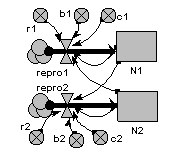# Lotka-Volterra two-species competition model

ModelId:
competition1
SimileVersion:
3.1+This is the classic textbook model of the population dynamics of two competing species.

It is based on a pair of differential equations, each having the same mathematical form:

dX1/dt = r1.X1.(1-b1.X1-c1.X2)
dX2/dt = r2.X2.(1-b2.X2-c2.X1)

where:
X1,X2 are the populations sizes of the two species;
r1,r2 are the intrinsic rate sof increase of the two species;
b1,b2 are the self-inhibition coefficients for the two species;
c1,c2 are the competitor’s inhibition coefficient for each species.

Results: